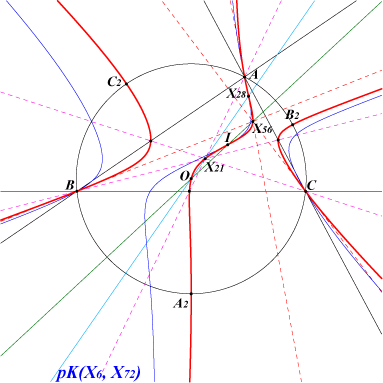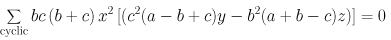X(1), X(3), X(21), X(28), X(56), X(58), X(84), X(1394), X(2360) A2, B2, C2 : vertices of CP2, the 2nd circumperp triangle (the tangents at these points are the perpendicular bisectors)K317 is the locus of point P whose cevian triangle is perspective to CP2, the 2nd circumperp triangle (see TCCT, §6.22). The locus of the perspector is K318. K318 is also the locus of point P whose antipedal triangle is perspective to CP1, the 1st circumperp triangle (see TCCT, §6.21) together with the line at infinity and the circumcircle, circumcevian triangle is perspective to TCP2, the tangential triangle of the 2nd circumperp triangle (see TCCT, §6.24) together with the line at infinity and the circumcircle. See Q173 for another locus property. See also table 6. K318 has the same asymptotic directions as pK(X6, X72). K318 is the isogonal transform of the Spieker central cubic K033 = pK(X37, X8). It is anharmonically equivalent to the Thomson cubic. See Table 21. K318 is a circumcevian pK. See CL072.